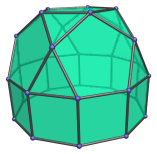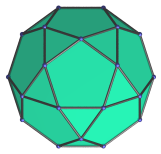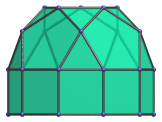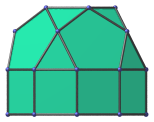# The Elongated Pentagonal Rotunda

The elongated pentagonal rotunda is the 21st Johnson solid (J21). It has 30 vertices, 55 edges, and 27 faces (10 equilateral triangles, 10 squares, 6 pentagons, and 1 decagon).The elongated pentagonal rotunda can be constructed by attaching a decagonal prism to a pentagonal rotunda (J6), lengthening it; hence the name elongated.

## Projections

Here are some views of the elongated pentagonal rotunda from various angles:

Projection Envelope DescriptionRegular decagon

Top view.Octagon

Front view.Heptagon

Side view.

## Coordinates

The Cartesian coordinates of the elongated pentagonal rotunda with edge length 2 are:

• (0, -√((10+2√5)/5), 1+√((20+8√5)/5))
• (±1, √((5+2√5)/5), 1+√((20+8√5)/5))
• (±φ, -√((5-√5)/10), 1+√((20+8√5)/5))
• (0, √((20+8√5)/5), 1+√((10+2√5)/5))
• (±φ, -√((25+11√5)/10), 1+√((10+2√5)/5))
• (±φ2, √((5+√5)/10), 1+√((10+2√5)/5))
• (±1, ±√(3+4φ), ±1)
• (±φ2, ±√(2+φ), ±1)
• (±2φ, 0, ±1)

where φ=(1+√5)/2 is the Golden Ratio.

Last updated 18 Jun 2019.